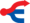# AND (BASIC)The title of this article is ambiguous. AND (Disambiguation).

 BASIC keyword Keyword: AND Abbreviation: A Shift+N Type: Operator Token code: 175/\$AF Handling routinein BASIC ROM: 45033–45077\$AFE9–B015 List of all BASIC keywords

Remark: This article describes the BASIC operator AND in BASIC V2 at the Commodore 64. For information on the assembler/machine language instruction of the same name, see AND (assembler).

```Type: Boolean/bitwise operator
General Programming-Syntax: <Boolean/integer term> AND <Boolean/integer term>
```

AND performs two different functions:

• In boolean expressions (such as the condition in an IF/THEN construct), AND yields the boolean value TRUE (numerical: −1) if, and only if, both of the terms preceding and trailing the AND keyword, evaluates to TRUE.
• In bitwise operations, a bit in the resulting integer is set if, and only if, the corresponding bits from both integer terms, are set.

In the case of bitwise AND operations, both terms are evaluated as 16-bit signed integers, and thus cannot exceed the range of −32768 thru 32767, or −\$8000 thru \$7FFF in hexadecimal — terms outside of this range will raise an ?ILLEGAL QUANTITY ERROR IN line. If either of the terms are absent, a ?SYNTAX ERROR IN line will occur.

Note that in BASIC on the C-64, the boolean value TRUE is represented by the integer −1, which is all bits set in the 16-bit signed format, while the boolean value FALSE is represented by the value 0, or all bits clear. Given that the equality (=) and inequality (<, > and <>) operators produce either −1 (TRUE) or 0 (FALSE) as numeric results, the AND keyword can be implemented as a bitwise, binary operator that performs both logical and bitwise functions the same way.

For instance, consider the following expression:

```PRINT "ABC"="ABC"
-1

```

As you can see, the equality operator (=) evaluates the two strings as a comparison and returns the numeric value TRUE (−1). Thus when two such true conditions are joined by the AND keyword, it evaluates them as a binary, bitwise operation of −1 AND −1, resulting in −1 (TRUE). Similarly, when one of the two operands is logically FALSE (0), then the binary bitwise operation of −1 AND 0 results in 0 (FALSE). Thus the logical and bitwise operations are, in fact, the same operation. Since AND is really only implemented once, as a binary, bitwise operator, the use of non-numeric operands in conjunction with AND causes a ?TYPE MISMATCH ERROR IN line.

For example:

```PRINT "ABC" AND "ABC"

?TYPE MISMATCH  ERROR
```

## Examples

```PRINT 3>2 AND 5<6
-1      meaning "true"

PRINT 2=2 AND 3<2
0      meaning "false"

PRINT 199 AND 64   Bitwise operation to
64                check if bit 26=64 is

POKE 53265,PEEK (53265) AND 247    Bitwise operation to turn off
a single bit in a register
```

Boolean algebra

```PRINT 0 AND 0 (Result: 0; false)
PRINT 1 AND 0 (Result: 0; false)
PRINT 0 AND 1 (Result: 0; false)
PRINT 1 AND 1 (Result: 1; true)
```
BASIC V2.0 (second release) Commands

ABS | AND | ASC | ATN | CHR\$ | CLOSE | CLR | CMD | CONT | COS | DATA | DEF | DIM | END | EXP | FN | FOR | FRE | GET | GET# | GOSUB | GOTO | IF | INPUT | INPUT# | INT | LEFT\$ | LEN | LET | LIST | LOAD | LOG | MID\$ | NEW | NEXT | NOT | ON | OPEN | OR | PEEK | π | POKE | POS | PRINT | PRINT# | READ | REM | RESTORE | RETURN | RIGHT\$ | RND | RUN | SAVE | SGN | SIN | SPC | SQR | STATUS/ST | STEP | STOP | STR\$ | SYS | TAB | TAN | THEN | TIME/TI | TIME\$/TI\$ | TO | USR | VAL | VERIFY | WAIT